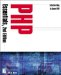# Operators

An operator is a symbol that represents a specific action. For example, the + arithmetic operator adds two values, and the = assignment operator assigns a value to a variable.

### Arithmetic Operators

Arithmetic operators bear a striking resemblance to simple math, as shown in Table A.2. In the examples, \$a = 5 and \$b = 4.

Table A.2: Arithmetic Operators

Operator

Name

Example

+

\$c = \$a + \$b; // \$c = 9

-

subtraction

\$c = \$a - \$b; // \$c = 1

*

multiplication

\$c = \$a * \$b; // \$c = 20

/

division

\$c = \$a / \$b; // \$c = 1.25

%

modulus, or "remainder"

\$c = \$a % \$b; // \$c = 1

### Assignment Operators

The = sign is the basic assignment operator:

` \$a = 124; // the value of \$a is 124 `

Other assignment operators include +=, -=, and .=.

` \$ex += 1; // Assigns the value of (\$ex + 1) to \$ex.                   // If \$ex = 2, then the value of (\$ex += 1) is 3. \$ex -= 1; // Assigns the value of (\$ex - 1) to \$ex.                   // If \$ex = 2, then the value of (\$ex -= 1) is 1. \$ex .= "coffee";  // Concatenates (adds to) a string. If \$ex = "I like " // then the value of (\$ex .= "coffee") is "I like coffee". `

### Comparison Operators

It should come as no surprise that comparison operators compare two values. A value of true or false is returned by the comparison, as shown in Table A.3.

Table A.3: Comparison Operators

Operator

Name

Example

Result (T/F)

==

equal to

\$a == \$b

TRUE if \$a is equal to \$b

!=

not equal to

\$a != \$b

TRUE if \$a is not equal to \$b

>

greater than

\$a > \$b

TRUE if \$a is greater than \$b

<

less than

\$a < \$b

TRUE if \$a is less than \$b

>=

greater than or equal to

\$a >= \$b

TRUE if \$a is greater than or equal to \$b

<=

less than or equal to

\$a <= \$b

TRUE if \$a is less than or equal to \$b

### Increment/Decrement Operators

The increment/decrement operators do just what their name implies: add or subtract from a variable (see Table A.4).

Table A.4: Increment/Decrement Operators

Name

Usage

Result

++\$a

Pre-increment

Increments by 1 and returns \$a

\$a++

Post-increment

Returns \$a and then increments \$a by 1

--\$a

Pre-decrement

Decrements by 1 and returns \$a

\$a--

Post-decrement

Returns \$a and then decrements \$a by 1

### Logical Operators

Logical operators allow your script to determine the status of conditions and, in the context of your if...else or while statements, execute certain code based on which conditions are true and which are false (see Table A.5).

Table A.5: Logical Operators

Operator

Name

Example

Result (T/F)

!

not

!\$a

TRUE if \$a is not true

&&

and

\$a && \$b

TRUE if both \$a and \$b are true

||

or

\$a || \$b

TRUE if either \$a or \$b is truePHP Essentials, 2nd Edition
ISBN: 1931841349
EAN: 2147483647
Year: 2002
Pages: 74